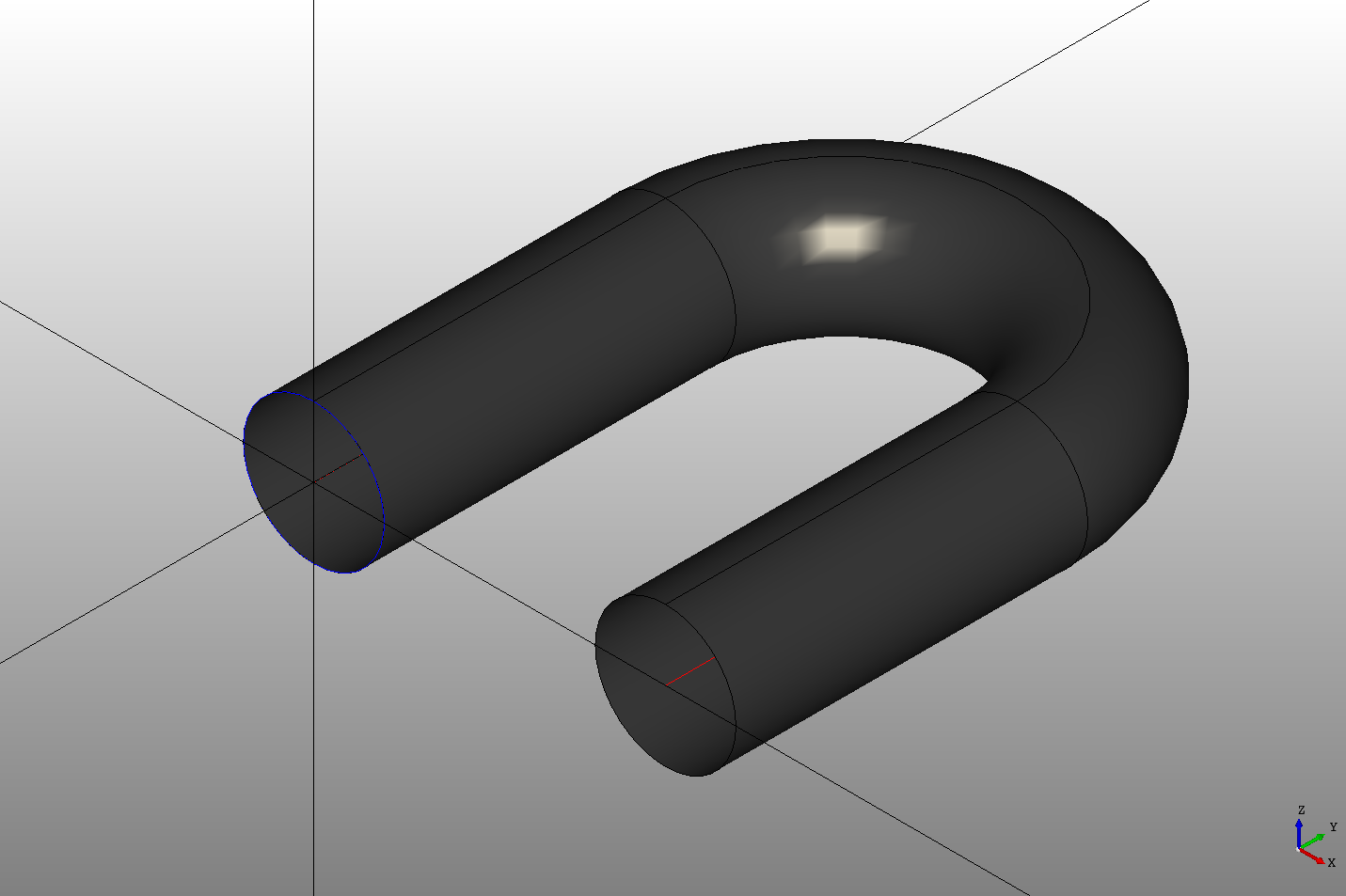## Sweeps

In DeclaraCAD to "sweep" a profile the `Pipe` declaration is used as it follows OpenCASCADE naming. The `Pipe` declaration requires two shapes to operate on, the `profile` and the `spline`.

Note: Add `from declaracad.occ.api import Pipe as Sweep` to rename if you prefer the name sweep instead

``````# Created in DeclaraCAD

enamldef Path(Wire):
Segment: s1:
points = [(0,0), (0,10)]
#: 180 deg elbow
Arc: a1:
attr p = s1.points[-1]
points = [
p,
]
Segment:
attr p = a1.start # Arc goes backwards
points = [p, (10, 0)]

enamldef Assembly(Part):
Path: path:
color = 'red'

Circle: pipe_profile:
color = 'blue'
direction = (0,1,0)

Pipe:
color = '#333'
spline = path
profile = pipe_profile
``````

Which creates the following pipe.Instead of assigning `spline` and `profile` attributes they may be added into the `Pipe` block, the first being the spline and the second being the profile for example:

``````enamldef Assembly(Part):
Pipe:
color = '#333'
Path: path:
pass
Circle: pipe_profile:
direction = (0,1,0)
``````

It will also accept one child declaration and one of the attributes.

## Alignment

It is important to note that the `spline` and `profile` being swept should start at the same position and the angle between the profile normal and spline direction is maintained throughout the sweep. Above the direction of the circle was set to the y axis since the first line segment moves in the y direction.

In some cases it may be hard to find the direction, in this case the position and direction of a curve can be obtained using the `get_value_at` method of the shapes topology. For example:

``````# Created in DeclaraCAD

enamldef Path(Wire):
alias s1
Segment: s1:
points = [(0,0), (0,10)]
Arc: a1:
attr p = s1.points[-1]
points = [
p,
]
Segment:
attr p = a1.start # Arc goes backwards
points = [p, (10, 0)]

enamldef Assembly(Part):
Pipe:
color = '#333'
Path: path:
color = 'red'

Circle: pipe_profile:
attr r = path.s1.get_value_at(t=0, derivative=1)
position = r
direction = r
As of this writing the you cannot get the derivative from the Wire itself so instead we add an `alias` to the first segment of the wire and use that.